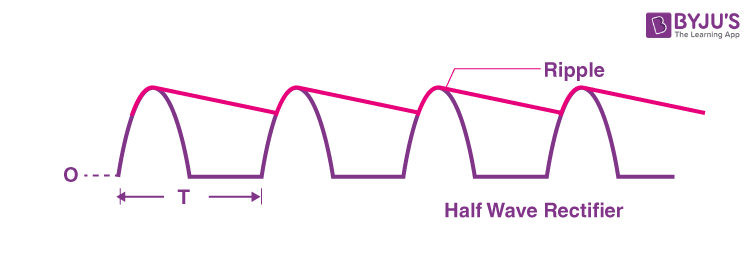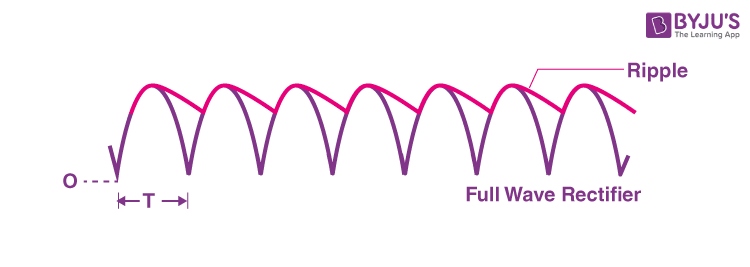# Ripple Factor

## What is Ripple Factor?

Ripple factor is defined as

The ratio of RMS value of an alternating current component in the rectified output to the average value of rectified output.

The ripple factor is denoted as γ. It is a dimensionless quantity and always has a value less than unity.

 Ripple factor, γ = RMS value of AC component in rectifier output/Average value of rectifier output
 γ = I’rms/Idc = V’rms/Vdc

In the above equation,

• I’rms is the alternating component of load current
• V’rms is the alternating component of voltage

### What is Ripple?

A ripple is defined as the fluctuating AC component in the rectified DC output.

The rectified DC output could be either DC current or DC voltage. When the fluctuating AC component is present in DC current it is known as the current ripple while the fluctuating AC component in DC voltage is known as the voltage ripple.

## Ripple Factor Formula

The ripple factor formula is defined in terms of the RMS value and the average value of the rectifier output. It is given as:

 $Ripple\;factor,\Gamma =\frac{\sqrt{(I_{rms})^{2}-(I_{dc})^{2}}}{I_{dc}}=\frac{\sqrt{(V_{rms})^{2}-(V_{dc})^{2}}}{V_{dc}}$

### Derivation of Ripple Factor Formula

From the definition of ripple factor, we know that there are two parameters that need to be determined:

• RMS value of the ripple present in either rectifier output current or output voltage.
• The average value of the output of the rectifier for one time period, T.

Ripple is given as the difference between the actual output and the expected DC output. The mathematical expression is:

Ripple = iL – Idc = vL – Vdc

Where,

• iL is the output current
• vL is the output voltage
• Idc is the average value of the load current
• Vdc is the average value of the load voltage

The RMS value of voltage ripple is given as:

$RMS\;value\;of\;ripple=\sqrt{\frac{1}{T}\int_{0}^{T}(i_{L}-I_{dc})^{2}d(\omega t)}$ $=\sqrt{\frac{1}{T}\int_{0}^{T}[(i_{L})^{2}+(I_{dc})^{2}-2(i_{L})(I_{dc})]d(\omega t)}$

However, we know that the effective value of the load current is given as:

$(I_{rms})=\sqrt{\frac{1}{T}\int_{0}^{T}(i_{L})^{2}d(\omega t)}$

Therefore,

RMS value of ripple is given as:

$=\sqrt{\frac{1}{T}\int_{0}^{T}[(i_{L})^{2}+(I_{dc})^{2}-2(i_{L})(I_{dc})]d(\omega t)}$ $=\sqrt{(I_{rms})^{2}+\frac{1}{T}\int_{0}^{T}[(I_{dc})^{2}-2(i_{L})(I_{dc})]d(\omega t)}$ $=\sqrt{(I_{rms})^{2}+\frac{1}{T}\int_{0}^{T}[(I_{dc})^{2}d(\omega t)-(\frac{2I_(dc)}{T})\int_{0}^{T}(i_{L})d(\omega t)}$

The average value is given as:

$I_{dc}=\sqrt{\frac{1}{T}\int_{0}^{T}(i_{L})d(\omega t)}$

The second integration will be equal to Idc

$RMS\;value\;of\;ripple=\sqrt{(I_{rms})^{2}+(\frac{1}{T})\int_{0}^{T}(I_{dc})^{2}d(\omega t)-2(I_{dc})^{2}}$ $=\sqrt{(I_{rms})^{2}+\frac{(I_{dc})^{2}}{T}[\omega t-0]-2(I_{dc})^{2}}$

But ωt = T

Therefore,

$RMS\;value\;of\;ripple=\sqrt{(I_{rms})^{2}+(I_{dc})^{2}-2(I_{dc})^{2}}$ $=\sqrt{(I_{rms})^{2}-(I_{dc})^{2}}$

We know that the ripple factor is equal to the ratio of RMS value and the average value of the rectifier output, which is given as:

$\Gamma =\frac{\sqrt{(I_{rms})^{2}-(I_{dc})^{2}}}{I_{dc}}$ $\Gamma =\frac{\sqrt{(V_{rms})^{2}-(V_{dc})^{2}}}{V_{dc}}$

### Ripple Factor of Half Wave Rectifier

From the formula of ripple factor, we know that

$\Gamma =\sqrt{(\frac{V_{rms}}{V_{dc}})^{2}-1}$

Rearranging the above equation, we get the ripple factor of half wave rectifier as:

$\Gamma =\frac{I^{2}_{rms}-I^{2}_{dc}}{I_{dc}}=1.21$### Ripple Factor of Full Wave Rectifier

From the formula of ripple factor, we know that

$\Gamma =\sqrt{(\frac{V_{rms}}{V_{dc}})^{2}-1}$

Substituting the values we get the ripple factor of full-wave rectifier as:

$\Gamma =\sqrt{(\frac{V_{rms}}{V_{dc}})^{2}-1}=0.48$### Ripple Factor of Bridge Rectifier

The ripple factor of bridge rectifier is the same as the ripple factor of the full-wave rectifier, that is:

$\Gamma =\sqrt{(\frac{V_{rms}}{V_{dc}})^{2}-1}=0.48$

## Frequently Asked Questions – FAQs

### Name the basic types of rectifiers.

There are three types of rectifiers and they are:

• Half-wave rectifier
• Full-wave centre-tap rectifier
• Full-wave bridge rectifier

### What is the difference between half-wave and full-wave rectifier?

The difference between half-wave and full-wave rectifier is that in half-wave rectifier only half of the AC cycle is converted whereas, in the full-wave rectifier, both the half cycles are converted.

### What is transformer utilization factor?

The transformer utilization factor is defined as the ratio of power delivered to the load and alternating current rating of the secondary supply power transformer.

### In rectifiers, diodes do not operate in the breakdown region. Why?

In rectifiers, diodes do not operate in the breakdown region because the diode has a high chance of getting burnt or damaged as there is an increase in the magnitude of the current flowing through the diode.

### What is the advantage of bridge rectifier over centre tapped full wave rectifier?

Following are the list of advantages of bridge rectifier over centre tapped full wave rectifier:

• The peak inverse voltage of a bridge rectifier is half of the centre-tapped rectifier.
• The transformer utilization factor of a bridge rectifier is 81.2% whereas a centre-tapped rectifier is 67.2%.
• A bridge rectifier doesn’t require a centre-tapped transformer.